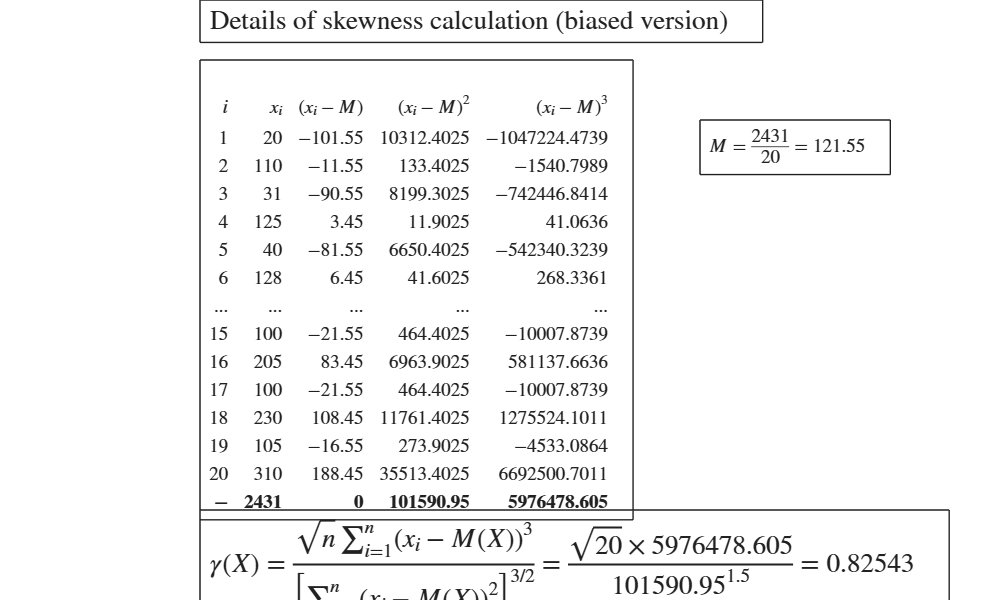# GUIskewness

GUIskewness shows the necessary calculations to obtain the variance in a GUI.

## Syntax

• out=GUIskewness(x)example
• out=GUIskewness(x, flag)example
• out=GUIskewness(x, flag, w)example

## Description

 out =GUIskewness(x) Calculation of biased index of skewness.

 out =GUIskewness(x, flag) Calculation of unbiased index of skewness.

 out =GUIskewness(x, flag, w) Calculation of weighted unbiased index of skewness.

## Examples

expand all

###Calculation of biased index of skewness.

Vector x contains the expenditure of 20 customers in a supermarket. (See page 178 of [MRZ])

x=[20, 110,  31, 125,  40, 128,  50, 140,  65, 150,  72, 175,...
85, 190,  100, 205,  100, 230,  105 310];
GUIskewness(x)
ans =

struct with fields:

data: [21×5 table]
gamma: 0.8254### Calculation of unbiased index of skewness.

Vector x contains the expenditure of 20 customers in a supermarket. (See page 178 of [MRZ])

x=[20, 110,  31, 125,  40, 128,  50, 140,  65, 150,  72, 175,...
85, 190,  100, 205,  100, 230,  105 310];
GUIskewness(x,0)

### Calculation of weighted unbiased index of skewness.

Italian grades obtained in a particular university exam by 1000 students. (See page 54 of [CMR])

x=20:29;
% w= vector of frequencies
w=[61    82   102   164   122   122    61   143    82    61];
GUIskewness(x,0,w)

## Related Examples

expand all

### Calculation of weighted biased index of skewness.

Italian grades obtained in a particular university exam by 1000 students. (See page 54 of [CMR])

x=20:29;
% w= vector of frequencies
w=[61    82   102   164   122   122    61   143    82    61];
GUIskewness(x,1,w)

## Input Arguments

### x — vector of numeric data. Vector.

Vector containing strictly numerical data.

Data Types: double

### flag — flag for unbiasness. Scalar.

If flag=0 the unbiased index is computed.

If flag=1 (default) the biased index is computed, meaning that it tends to differ from the population skewness by a systematic amount based on the sample size.

Example: 1 

Data Types: double

### w — weights. Vector.

Vector of the same length of x containing the weights assigned to each observation.

Example: 1:10 

Data Types: double

## Output Arguments

### out —detailed output to compute the index. Table

Table with n+1 rows (where n is the length of x) containing what is shown in the GUI. Last row contains the totals.

Milioli, M.A., Riani, M., Zani, S. (2019), "Introduzione all'analisi dei dati statistici (Quarta edizione ampliata)". [MRZ]

Cerioli, A., Milioli, M.A., Riani, M. (2016), "Esercizi di statistica (Quinta edizione)". [CMR]